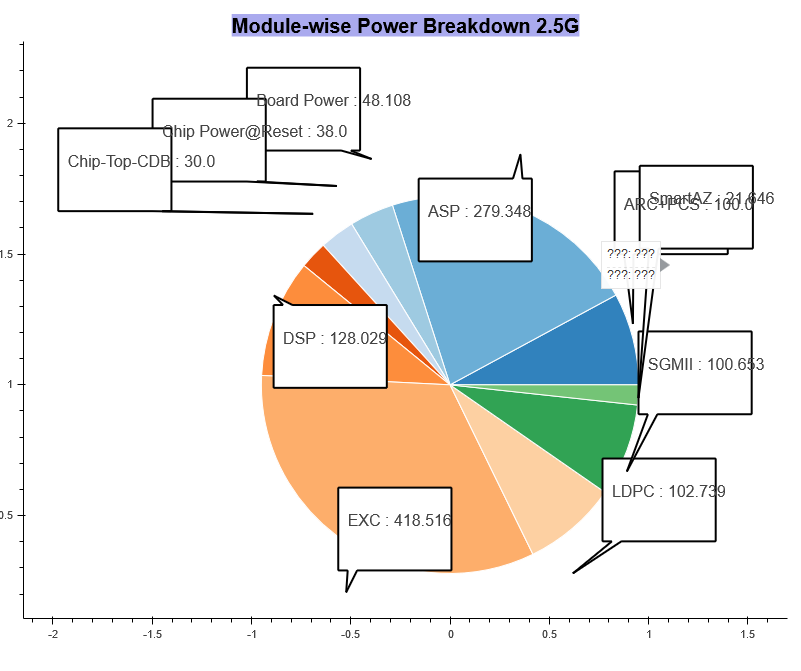# problem with wedge

hello,

I am trying to add some polygons to the wedge but I got a problem

when I calculate the position of the edge of the circle I get wrong coodinates and the circle seem to be confusing it doesn’t follow the y axis the radius in my case is 0.95 but in the figure it is around 0.7 in the y axis and 0.95 in the x axisthis is how I am getting the edge coordinates

ang = 0
x =
y =
for d in df:
##d is the angle and ang is the cumulative angle
x.append((radius - text_offset) * cos(ang + d/2))
y.append((radius - text_offset) * sin(ang + d/2) + 1)

``````    ang = ang + d
return x, y
``````

``

Hi,

The low level canvas primitives that draw circles/wedges always draw physical circles (i.e. circular in terms of square pixels). That means if the "data" aspect ratio of your x- and y- ranges does not match up with pixel aspect ratio of the plot on the screen, you get the situation you describe, where the radius does not match up in both dimensions. To deal with this, you should pass:

match_aspect=True

To your call to "figure". This will cause Bokeh to automatically adjust the x- and y- data range extents so that the data and screen aspect ratios are always matched (i.e. so circles measure the same radius in all directions). Note that you have to use default "auto ranging" for this to function. If you explicitly set range start/end yourself, bokeh assumes you know what you are doing and know what you want, and will use your values as given (i.e. it will ignore match_aspect if you set your own values).

Thanks,

Bryan

···

On Dec 15, 2018, at 03:10, [email protected] wrote:

hello,

I am trying to add some polygons to the wedge but I got a problem

when I calculate the position of the edge of the circle I get wrong coodinates and the circle seem to be confusing it doesn't follow the y axis the radius in my case is 0.95 but in the figure it is around 0.7 in the y axis and 0.95 in the x axis

<bokeh.png>

this is how I am getting the edge coordinates

ang = 0
x =
y =
for d in df:
##d is the angle and ang is the cumulative angle
x.append((radius - text_offset) * cos(ang + d/2))
y.append((radius - text_offset) * sin(ang + d/2) + 1)

ang = ang + d
return x, y

--
You received this message because you are subscribed to the Google Groups "Bokeh Discussion - Public" group.
To unsubscribe from this group and stop receiving emails from it, send an email to [email protected].
To post to this group, send email to [email protected].
To view this discussion on the web visit https://groups.google.com/a/continuum.io/d/msgid/bokeh/91d6dd9d-e688-4250-8c58-be20be0496e8%40continuum.io.